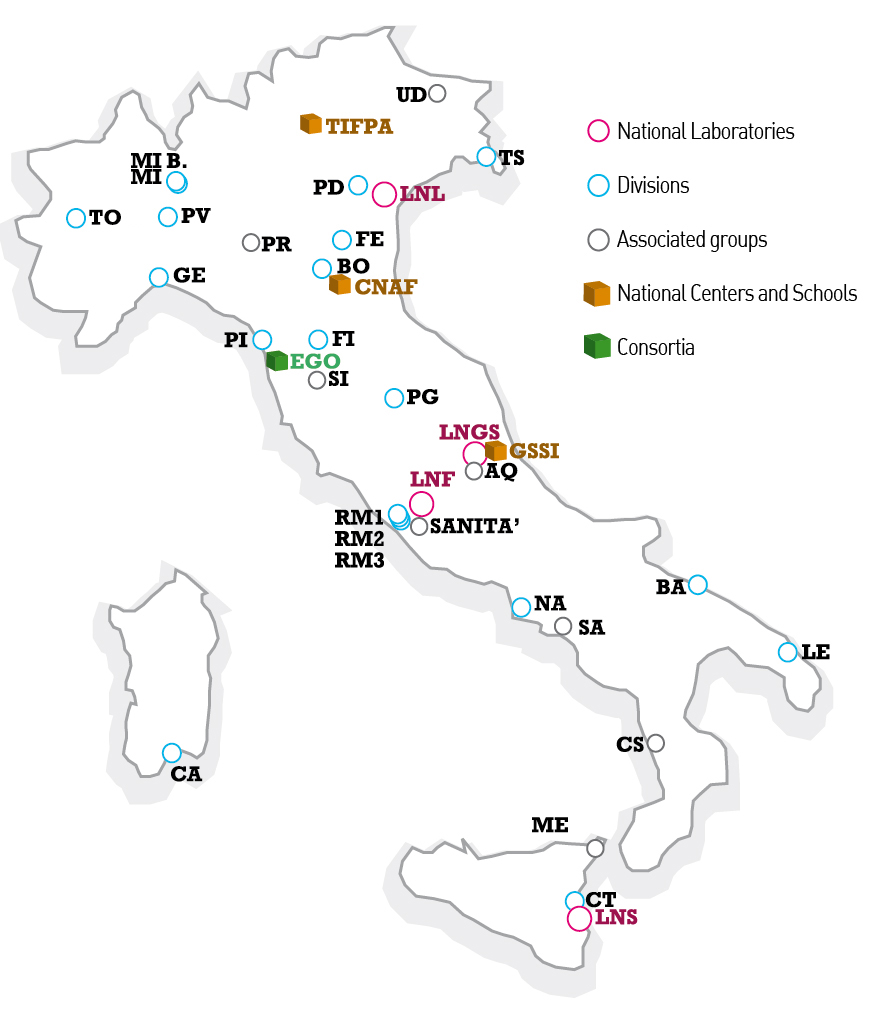# Strong interactions in the Standard Model and beyond and lattice field theory

### Abstract

The main objective of this project is to search for new fundamental phenomena in Nature by advancing the theoretical knowledge on strongly interacting theories in the Standard Model (SM) and beyond.

We strive for developing new theoretical and computational tools in order to carry out high precision  studies of the strong dynamics interactions by employing the first principles lattice field theory setup.  Our aim is to achieve computations with precision at the level of percent or less in order to match the accuracy required for the interpretation and the analysis of the wealth of experimental results expected both for sub-nuclear matter and for the quark-gluon plasma.

The project is organized in four groups of objectives as follows:

- QCD and flavor physics in the SM and beyond: determination of the hadronic contribution to (g − 2)μ at a few per mille level with multi-level Monte Carlo, precise determination of fundamental SM parameters, weak matrix elements for leptonic, semi-leptonic and ΔS = 2 transitions.

- QCD at high temperature and finite density: study of the phase diagram in the temperature - chemical  potential  plane, precise determination of the Equation of State (EoS) and light screening masses of QCD at chemical potential μ=0 up to temperatures of O(100) GeV, temperature dependence of the axion potential.

- Theoretical developments: non-perturbative renormalization of fundamental parameters and composite operators, Lefschetz thimbles, chiral symmetry breaking.

- Computational strategies: fermion determinant factorization, multi-level Monte Carlo, Lefschetz thimbles, master field simulations, numerical stochastic perturbation theory.

### Map of INFN facilities